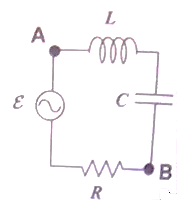# Problem: In the circuit shown below, the AC generator supplies an EMF of the form ε = 15sin (100t - π/3) volts. A student measures the current to be I = 6 sin(100t) amps. Thus, the generator voltage lags the current by π/3 radian (i.e. 60°). The average power delivered by the AC generator is:a. 22.5 Wb. 45.0 Wc. 39.0 W

###### Problem Details

In the circuit shown below, the AC generator supplies an EMF of the form ε = 15sin (100t - π/3) volts. A student measures the current to be I = 6 sin(100t) amps. Thus, the generator voltage lags the current by π/3 radian (i.e. 60°). The average power delivered by the AC generator is:

a. 22.5 W

b. 45.0 W

c. 39.0 W